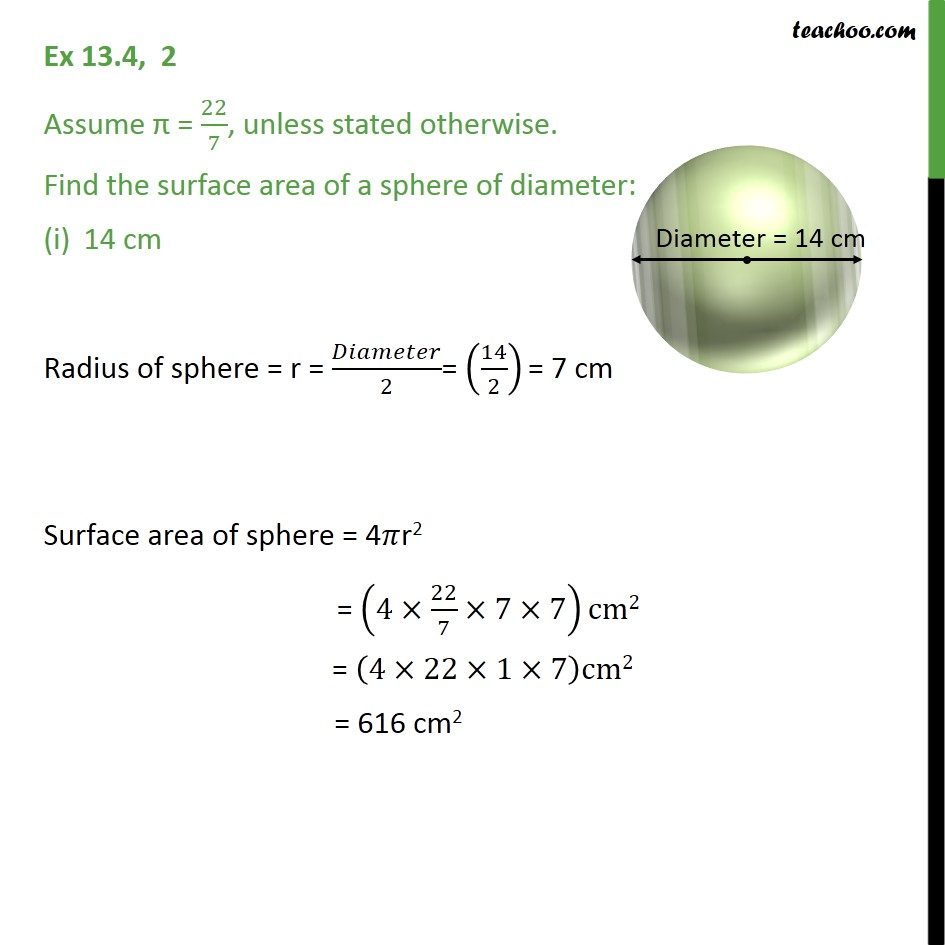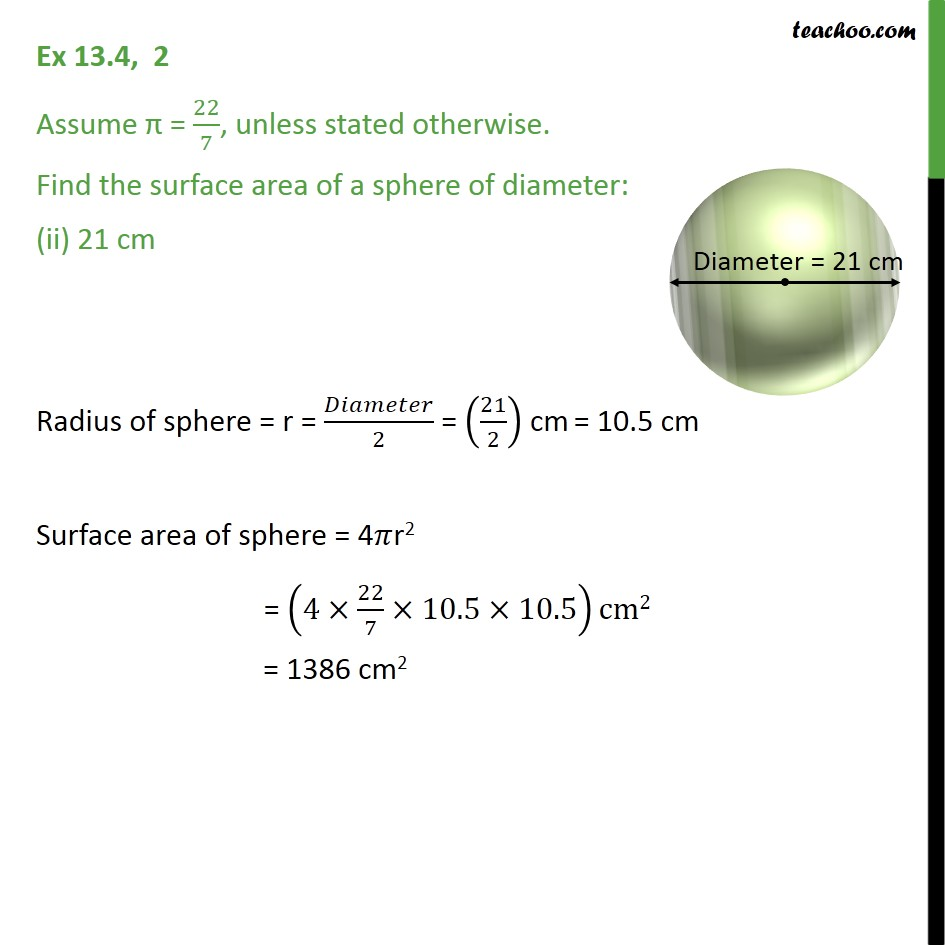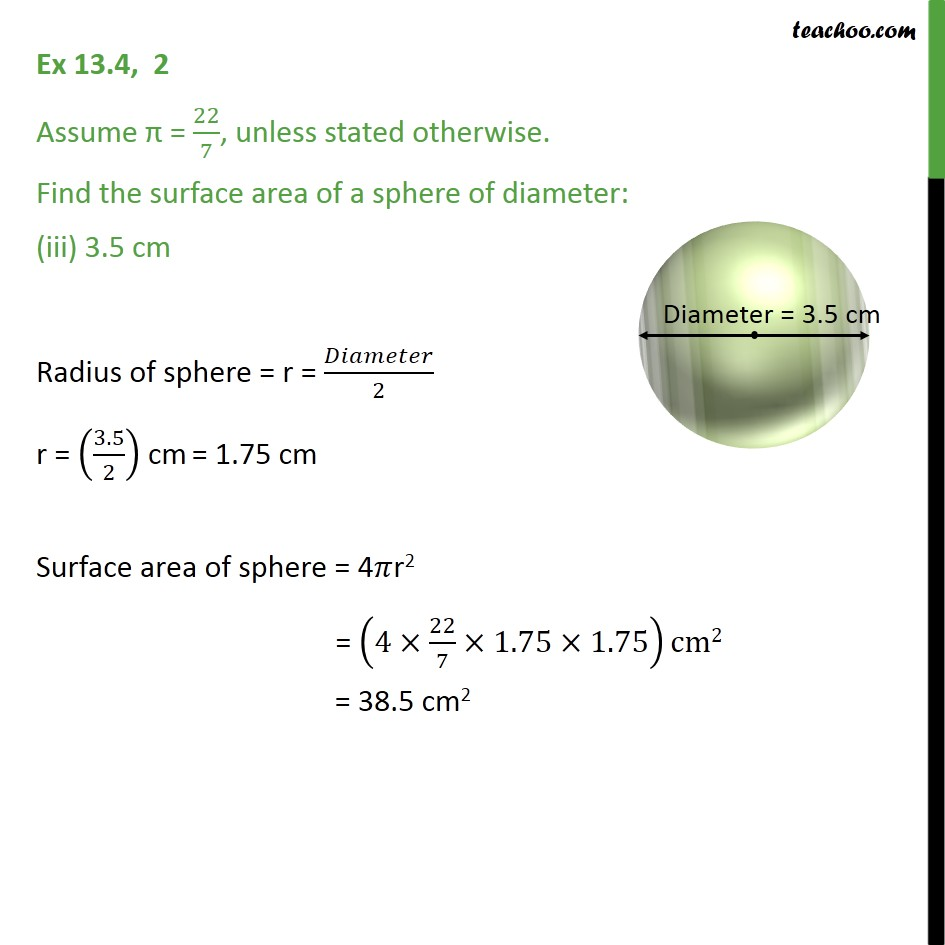Subscribe to our Youtube Channel - https://you.tube/teachoo

1. Chapter 13 Class 9 Surface Areas and Volumes
2. Serial order wise
3. Ex 13.4

Transcript

Ex 13.4, 2 Assume = 22/7, unless stated otherwise. Find the surface area of a sphere of diameter: 14 cm Radius of sphere = r = /2= (14/2) = 7 cm Surface area of sphere = 4 r2 = (4 22/7 7 7)cm2 = (4 22 1 7)cm2 = 616 cm2 Ex 13.4, 2 Assume = 22/7, unless stated otherwise. Find the surface area of a sphere of diameter: (ii) 21 cm Radius of sphere = r = /2 = (21/2) cm = 10.5 cm Surface area of sphere = 4 r2 = (4 22/7 10.5 10.5)cm2 = 1386 cm2 Ex 13.4, 2 Assume = 22/7, unless stated otherwise. Find the surface area of a sphere of diameter: (iii) 3.5 cm Radius of sphere = r = /2 r = (3.5/2) cm = 1.75 cm Surface area of sphere = 4 r2 = (4 22/7 1.75 1.75)cm2 = 38.5 cm2

Ex 13.4